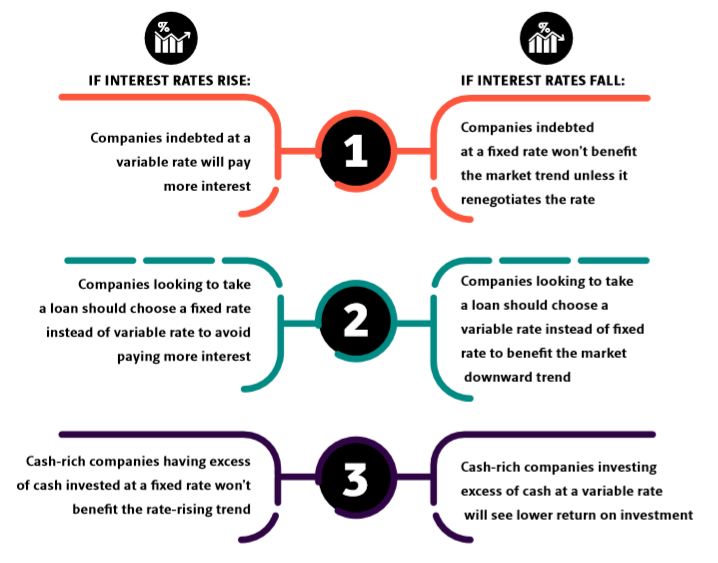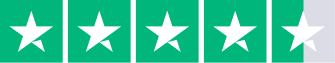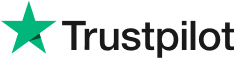### Contents

Does interest rate risk have the same weight as FX risk? Interest rates are usually far less volatile than FX rates, therefore a company’s FX exposure is usually greater than its interest rate exposure. However, the latter must not be ignored, as interest rate fluctuations can be as unpredictable as FX rate changes.

## What is the impact of interest rate volatility on a company?

The infographic below summarizes the interest rate risk in six scenarios: